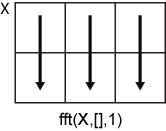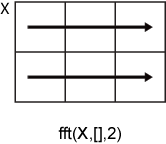# fft

## 语法

``Y = fft(X)``
``Y = fft(X,n)``
``Y = fft(X,n,dim)``

## 说明

````Y = fft(X)` 用快速傅里叶变换 (FFT) 算法计算 `X` 的离散傅里叶变换 (DFT)。 如果 `X` 是向量，则 `fft(X)` 返回该向量的傅里叶变换。如果 `X` 是矩阵，则 `fft(X)` 将 `X` 的各列视为向量，并返回每列的傅里叶变换。如果 `X` 是一个多维数组，则 `fft(X)` 将沿大小不等于 1 的第一个数组维度的值视为向量，并返回每个向量的傅里叶变换。 ```

````Y = fft(X,n)` 返回 `n` 点 DFT。如果未指定任何值，则 `Y` 的大小与 `X` 相同。 如果 `X` 是向量且 `X` 的长度小于 `n`，则为 `X` 补上尾零以达到长度 `n`。如果 `X` 是向量且 `X` 的长度大于 `n`，则对 `X` 进行截断以达到长度 `n`。如果 `X` 是矩阵，则每列的处理与在向量情况下相同。如果 `X` 为多维数组，则大小不等于 1 的第一个数组维度的处理与在向量情况下相同。 ```

``Y = fft(X,n,dim)` 返回沿维度 `dim` 的傅里叶变换。例如，如果 `X` 是矩阵，则 `fft(X,n,2)` 返回每行的 n 点傅里叶变换。`

## 示例

```Fs = 1000; % Sampling frequency T = 1/Fs; % Sampling period L = 1500; % Length of signal t = (0:L-1)*T; % Time vector```

`S = 0.7*sin(2*pi*50*t) + sin(2*pi*120*t);`

`X = S + 2*randn(size(t));`

```plot(1000*t(1:50),X(1:50)) title('Signal Corrupted with Zero-Mean Random Noise') xlabel('t (milliseconds)') ylabel('X(t)')````Y = fft(X);`

```P2 = abs(Y/L); P1 = P2(1:L/2+1); P1(2:end-1) = 2*P1(2:end-1);```

```f = Fs*(0:(L/2))/L; plot(f,P1) title('Single-Sided Amplitude Spectrum of X(t)') xlabel('f (Hz)') ylabel('|P1(f)|')``````Y = fft(S); P2 = abs(Y/L); P1 = P2(1:L/2+1); P1(2:end-1) = 2*P1(2:end-1); plot(f,P1) title('Single-Sided Amplitude Spectrum of S(t)') xlabel('f (Hz)') ylabel('|P1(f)|')``````Fs = 100; % Sampling frequency t = -0.5:1/Fs:0.5; % Time vector L = length(t); % Signal length X = 1/(4*sqrt(2*pi*0.01))*(exp(-t.^2/(2*0.01)));```

```plot(t,X) title('Gaussian Pulse in Time Domain') xlabel('Time (t)') ylabel('X(t)')````n = 2^nextpow2(L);`

`Y = fft(X,n);`

```f = Fs*(0:(n/2))/n; P = abs(Y/n).^2; plot(f,P(1:n/2+1)) title('Gaussian Pulse in Frequency Domain') xlabel('Frequency (f)') ylabel('|P(f)|^2')``````Fs = 1000; % Sampling frequency T = 1/Fs; % Sampling period L = 1000; % Length of signal t = (0:L-1)*T; % Time vector```

```x1 = cos(2*pi*50*t); % First row wave x2 = cos(2*pi*150*t); % Second row wave x3 = cos(2*pi*300*t); % Third row wave X = [x1; x2; x3];```

```for i = 1:3 subplot(3,1,i) plot(t(1:100),X(i,1:100)) title(['Row ',num2str(i),' in the Time Domain']) end````n = 2^nextpow2(L);`

`dim = 2;`

`Y = fft(X,n,dim);`

```P2 = abs(Y/L); P1 = P2(:,1:n/2+1); P1(:,2:end-1) = 2*P1(:,2:end-1);```

```for i=1:3 subplot(3,1,i) plot(0:(Fs/n):(Fs/2-Fs/n),P1(i,1:n/2)) title(['Row ',num2str(i),' in the Frequency Domain']) end```## 输入参数

• `fft(X,[],1)` 沿 `X` 的各列进行运算，并返回每列的傅里叶变换。• `fft(X,[],2)` 沿 `X` 的各行进行运算，并返回每行的傅里叶变换。## 输出参数

`Y` 的大小如下：

• 对于 `Y = fft(X)``Y = fft(X,[],dim)``Y` 的大小等于 `X` 的大小。

• 对于 `Y = fft(X,n,dim)``size(Y,dim)` 的值等于 `n`，而所有其他维度的大小保持与在 `X` 中相同。

## 详细信息

### 向量的离散傅里叶变换

`Y = fft(X)``X = ifft(Y)` 分别实现傅里叶变换和傅里叶逆变换。对于长度为 `n``X``Y`，这些变换定义如下：

`$\begin{array}{l}Y\left(k\right)=\sum _{j=1}^{n}X\left(j\right)\text{\hspace{0.17em}}{W}_{n}^{\left(j-1\right)\text{​}\left(k-1\right)}\\ X\left(j\right)=\frac{1}{n}\sum _{k=1}^{n}Y\left(k\right)\text{\hspace{0.17em}}{W}_{n}{}^{-\left(j-1\right)\text{​}\left(k-1\right)},\end{array}$`

`${W}_{n}={e}^{\left(-2\pi i\right)/n}$`

## 提示

• `fft` 的执行时间取决于变换的长度。仅具有小质因数的变换长度的 fft 执行时间明显快于本身是质数或具有较大质因数的变换长度的 fft 执行时间。

• 对于大多数 `n` 值，实数输入的 DFT 需要的计算时间大致是复数输入的 DFT 计算时间的一半。但是，当 `n` 有较大的质因数时，速度很少有差别或没有差别。

• 使用工具函数 `fftw` 可能会提高 `fft` 的速度。此函数控制用于计算特殊大小和维度的 FFT 算法优化。

## 算法

FFT 函数（`fft``fft2``fftn``ifft``ifft2``ifftn`）基于一个称为 FFTW   的库。

 Frigo, M., and S. G. Johnson. “FFTW: An Adaptive Software Architecture for the FFT.” Proceedings of the International Conference on Acoustics, Speech, and Signal Processing. Vol. 3, 1998, pp. 1381-1384.